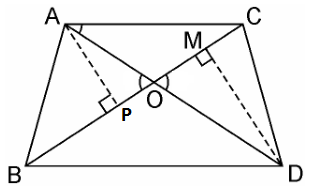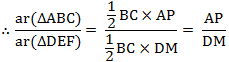Guru

# In the figure, ABC and DBC are two triangles on the same base BC. If AD intersects BC at O, show that area (ΔABC)/area (ΔDBC) = AO/DO. Q.3

• 0

How i solve the problem of class 10th of triangles of exercise 6.4 of class 10th math. Sir please help me for solving this problem In the figure, ABC and DBC are two triangles on the same base BC. If AD intersects BC at O, show that area (ΔABC)/area (ΔDBC) = AO/DO.

Share

1. Given, ABC and DBC are two triangles on the same base BC. AD intersects BC at O.

We have to prove: Area (ΔABC)/Area (ΔDBC) = AO/DO

Let us draw two perpendiculars AP and DM on line BC.We know that area of a triangle = 1/2 × Base × HeightIn ΔAPO and ΔDMO,

∠APO = ∠DMO (Each 90°)

∠AOP = ∠DOM (Vertically opposite angles)

∴ ΔAPO ~ ΔDMO (AA similarity criterion)

∴ AP/DM = AO/DO

⇒ Area (ΔABC)/Area (ΔDBC) = AO/DO.

• 0